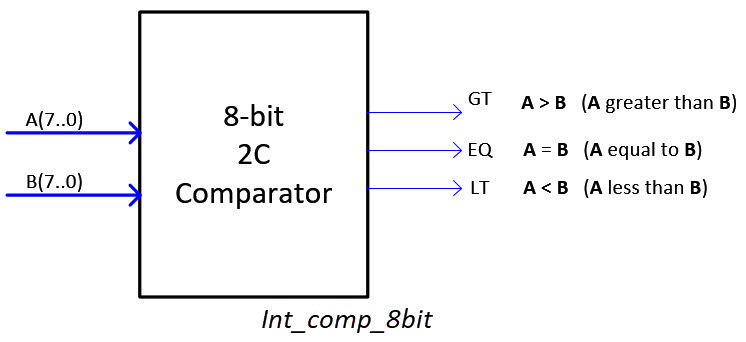﻿ Digital Circuits and Systems - Circuits i Sistemes Digitals (CSD) - EETAC - UPCBachelor's Degree in Telecommunications Systems and in Network EngineeringLecture 1 L4.1: Integer arithmetic in 2C [P4] Two's complement (2C) arithmetic for signed integer numbers [21/3]

1.10. Integer arithmetic

Let us start with symbols because they are an important tool to clarify what is expected from an arithmetic circuit and what data it will use.Typical symbols for integer (signed) numbers.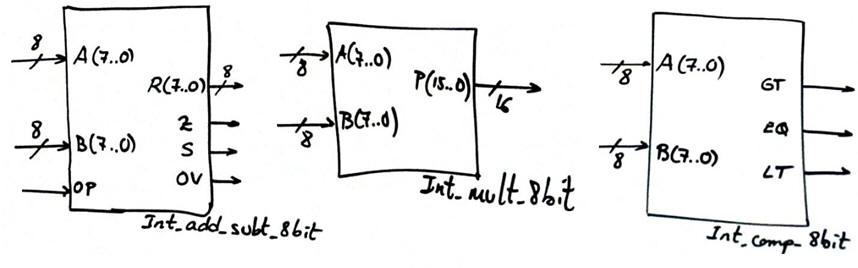Typical circuits that can operate two data types: when N = '0' unsigned (radix-2) and when N = '1' signed integers.1.10.1. Integer number two's complement (2C) representation

The key point is how to represent positive/negative numbers (integers): two's complement (2C) convention and range of values for a given number of N bits.

Represent several positive and negative numbers in two's complement and operate additions and subtractions.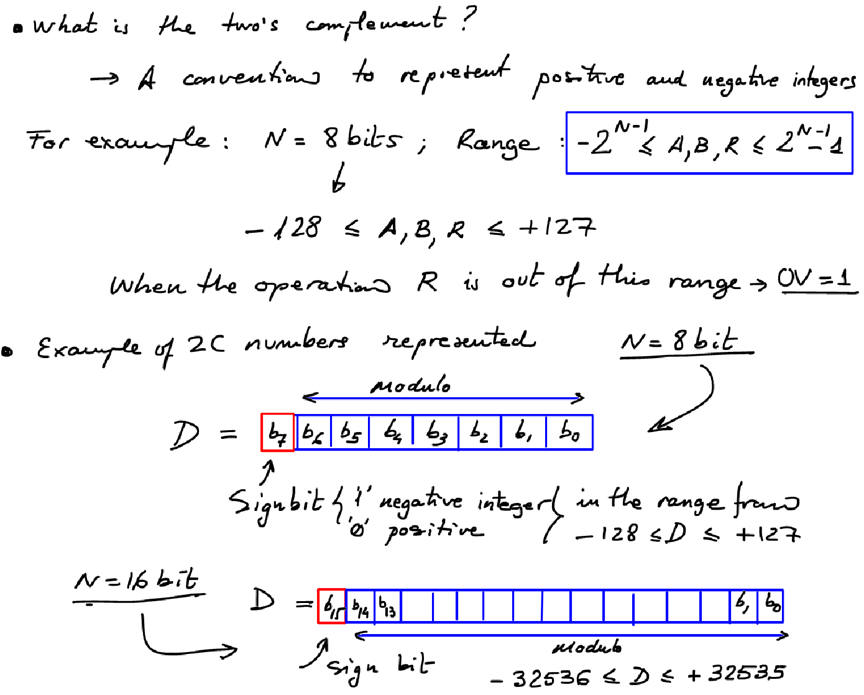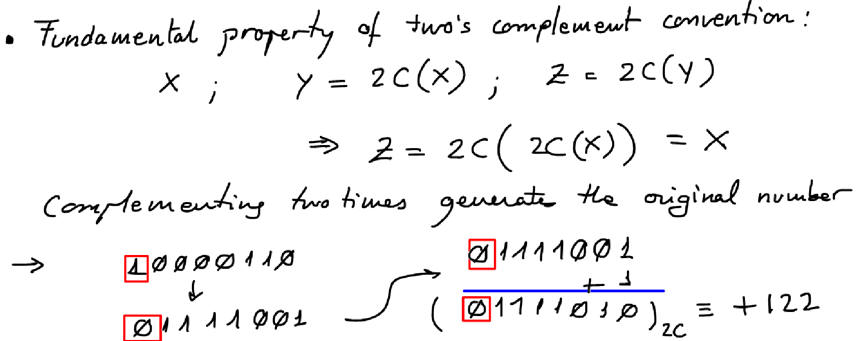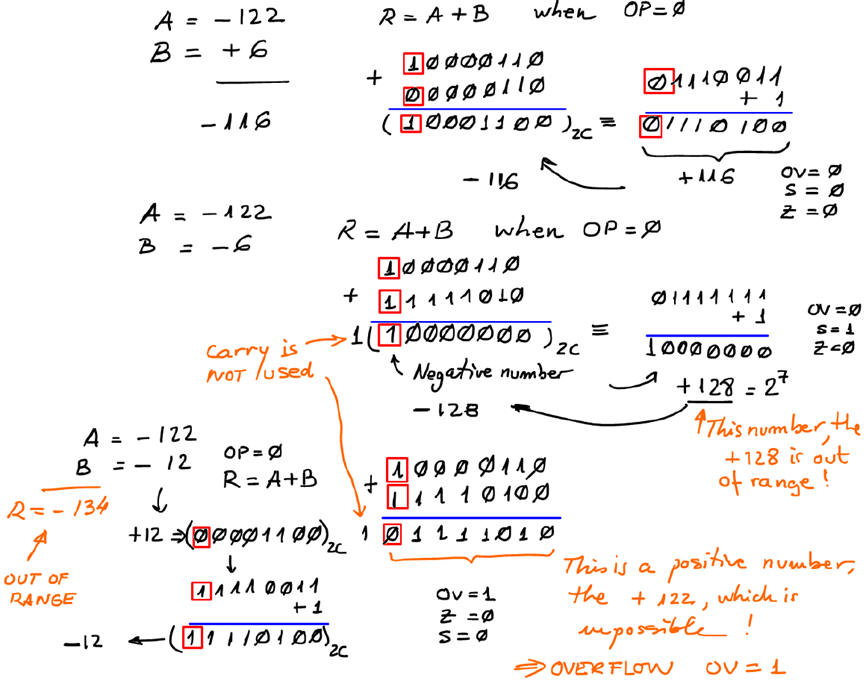Subtractions in 2C: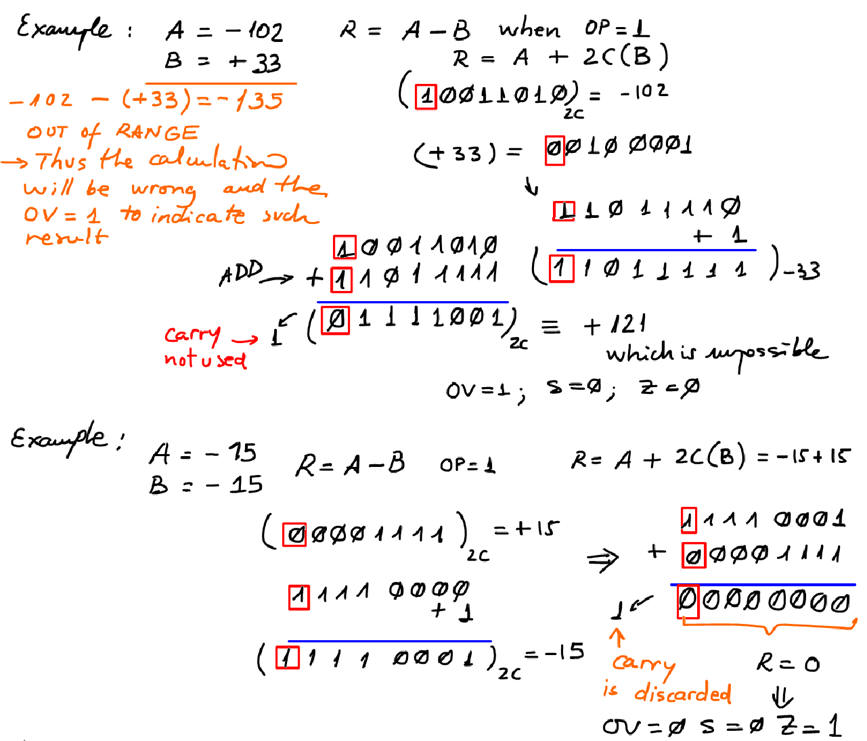Play with the 8-bit 2C adder and subtractor Int_add_subt_8bit.pdsprj available in Proteus.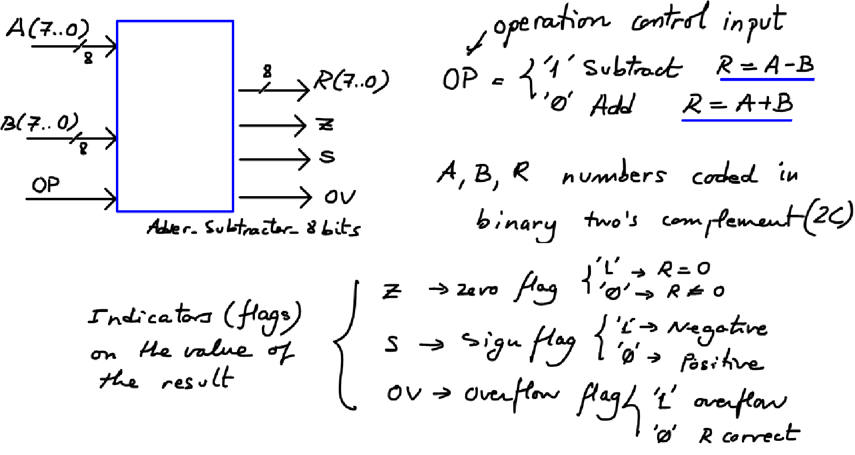Ideas on planning the circuit. The first idea that comes in mind is selecting (using a MUX) the outputs from two operators that work in parallel generating both operations concurrently. An Octuple_MUX_2 device functionality is visualised in the picture.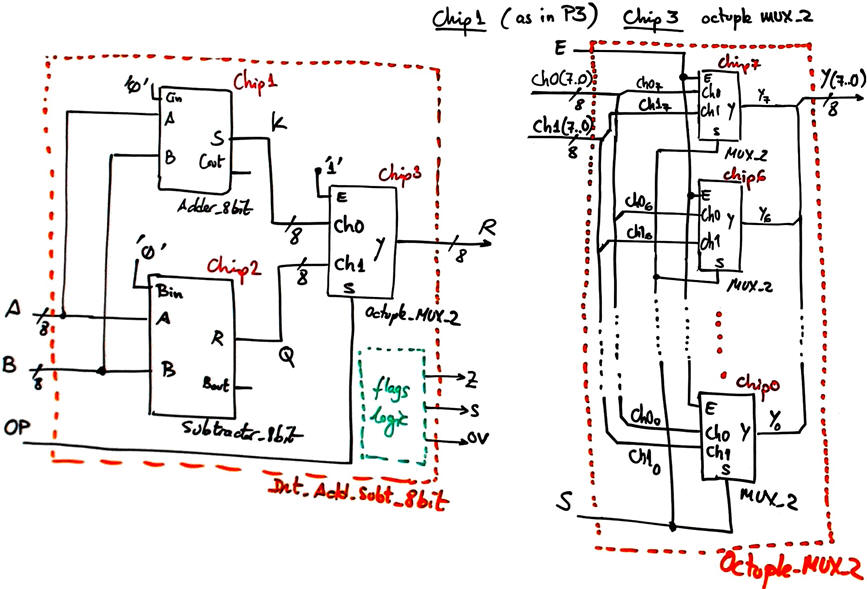Substractors can be inferred from specialised circuitry (see ) or taking advantage of two's complement convention.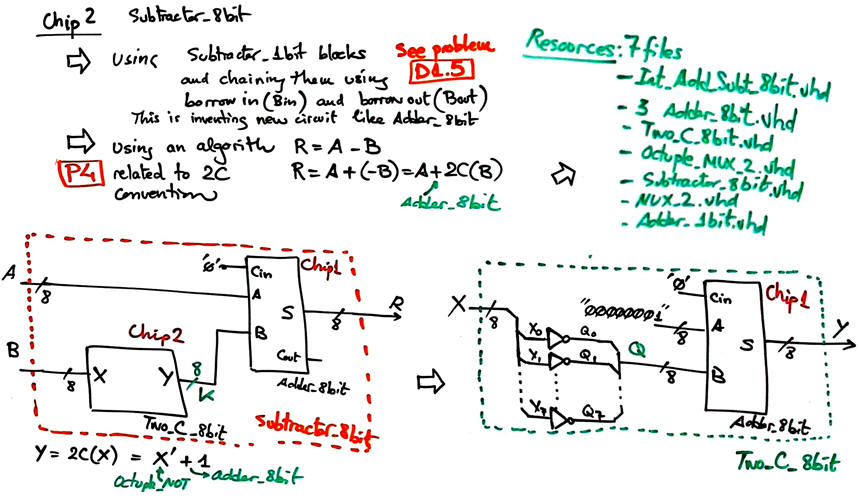However, instead of implementing the circuit above, this time we can easily optimise the architecture using only one Adder_8bit, as developed in detail in Int_Add_Subt_8bit circuit. Further details on how to calculate flags are also explained.

1.10.3. Multiplier (Selectable_ Mult_8bit)

How to design a multiplier for integer numbers using radix-2 multipliers?

This is an example of an structural hierarchical 8-bit selectable multiplier Selectable_mult_8bit.pdsprj built in Proteus using our unsigned Mult_8bit from P3 and other blocks to organise the sign algorithm for integer numbers. The circuit inlcudes an input N that allows the selection of data to be radix 2 or integer (in 2C).This problem is interesting because puts at the limits our hierarchical multiple-file plan (at this introductory level that does not include generic VHDL statement). The networks of components is too complex to be described in a text file. Optional hint: if this problem has to be solved in VHDL using EDA tools, perhaps is better to describe the Mult_8bit using a behavioural approach plan B instead of this complicated plan C2 architecture.

1.10.4. Comparator (Int_Comp_8_bit, etc.)

How to design a comparator for integer numbers using radix-2 comparators?. The idea is explored at .

Other basic operations in 2C: Comparison. The circuit Int_comp_8bit has to include 3 outputs: GT (greater than) to detect when A > B, EQ (equal to) to detect when A = B, and LT (less than) to detect when A < B.